# Electronics and Communication Engineering - Exam Questions Papers

26.

The electric field of uniform plane wave is given by E = 20 sin (2 p x 108t- pz)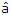x + 20 cos (2 p x 108t - pz)y. The polarization of wave is

 A. Elliptical, left handed B. Circular, left handed C. Elliptical, right handed D. Circular, right handed

Explanation:

The given two components are equal in amplitude, perpendicular and out of phase by 90°.

The wave is circularly polarized.

Now we consider z = 0 and put t = 0 and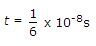At t = 0, E = 10ay

At t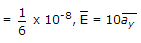Left circularly polarized.

27.

A two port network shown below is external dc sources. The voltages and the currents are measured with voltmeters V1 V2 and ammeter A1 A2 (all assumed to be ideal), as indicated. Under following switch conditions, the readings obtained are:
(i) S1 - Open, S2 - Closed A1 = 0A, V1 = 4.5 V, V2 = 1.5 V, A2 = 1 A
(ii) S1 - Closed, S2 - Open A1 = 4A, V1 = 6 V, V2 = 6 V, A2 = 0 A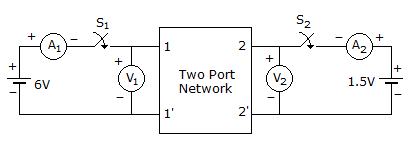The z-parameter matrix for this network is

 A.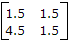B.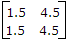C.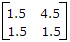D.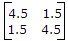Explanation:

V1 = Z11I1 + Z12I2

V2 = Z22I + Z22I2

Using the given information; S1-open, S2-closed

4.5 = Z121 ⇒Z12 = 4.5

1.5 = Z221 ⇒ Z22 = 1.5

S1-closed, S2-open

6 = Z111 ⇒ Z11 = 1.5

6 = Z214 ⇒ Z21 = 1.5

Thus Z matrix =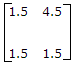28.

The Z-transform of a particular signal is given as X(Z) =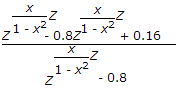where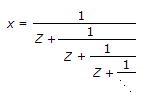This system after practical implementation will be

 A. Non Causal and Stable B. Causal and Stable C. Non Causal and Unstable D. Causal and Unstable

Explanation:

Let x =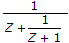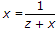zx + x2 = 1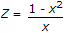Substitute in the equation given, we get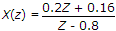Hence we have pole at Z = 0.8 for stability the ROC should be away from Z = 0.8 towards infinity.

29.

The JFET in the circuit shown in figure has an IDSS = 10 mA and Vp = -5 V. The value of the resistance Rs for a drain current IDS = 6.4 mA is (select the nearest value)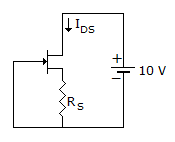A. 150 Ω B. 150 Ω C. 560 Ω D. 1 kΩ

Explanation: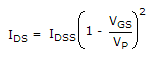6.4 x 10-3 = 10 x 10-3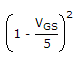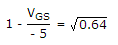= 0.8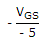= 0.8 - 1 = - 0.2

VGS = -5 x 0.2 = -1 V

IDSRS = -VGS = - (-1) = 1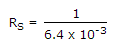= 0.156 x 103 = 156 Ω.

30.

In the circuit s closed for a long time and steady state is reached. s is opened at t = 0+ Determine current through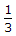resistor.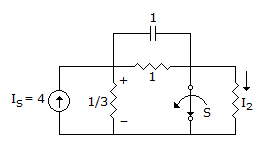A.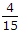A B.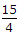A C. -A D. -A

Explanation:

Steady state with s closed.

C is open Is = 4.

This source current is divided between two resistorsΩ and 1 Ω.

Across 1 Ω, C is open, I in 1 Ω is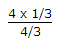= 1 A.

Hence voltage across C is 1 V. The initial charge on C is V0 = 1 V.

At t = 0+, s is opened bringing R = 1 Ω into the circuit.

C is replaced by 1 V source with positive polarity left.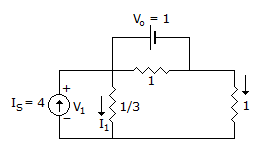The circuit has two sources Is = 4 and V0 = 1.

The currents in the resistor R =and R = 1 can be determined by superposition.

For R =Due to Is only (short V0)

I = Is x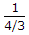= 3

Due to V0 only (open Is)

I =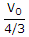=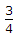Downwards

Adding, current through R =is 3=.

#### Current Affairs 2022

Interview Questions and Answers